# doc IEEE 802 11 180452 r 0 March

• Slides: 11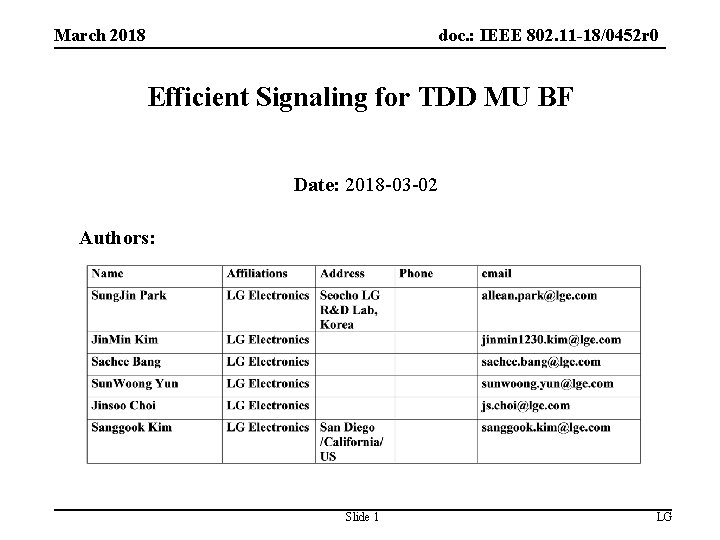doc. : IEEE 802. 11 -18/0452 r 0 March 2018 Efficient Signaling for TDD MU BF Date: 2018 -03 -02 Authors: Slide 1 LG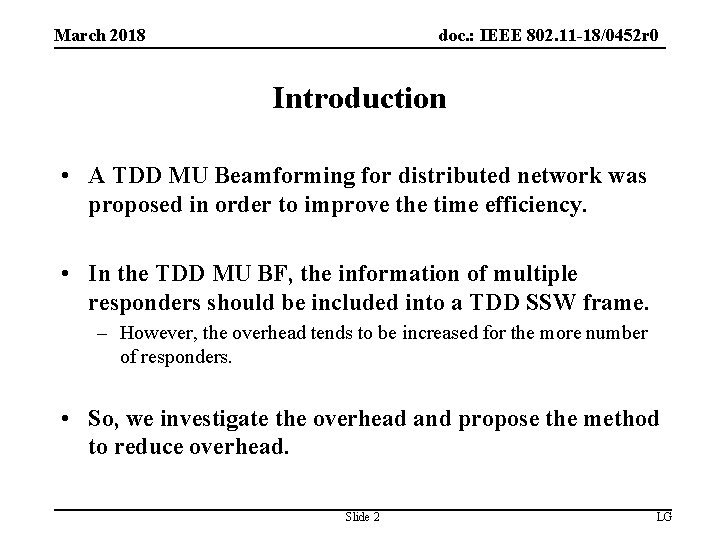doc. : IEEE 802. 11 -18/0452 r 0 March 2018 Introduction • A TDD MU Beamforming for distributed network was proposed in order to improve the time efficiency. • In the TDD MU BF, the information of multiple responders should be included into a TDD SSW frame. – However, the overhead tends to be increased for the more number of responders. • So, we investigate the overhead and propose the method to reduce overhead. Slide 2 LG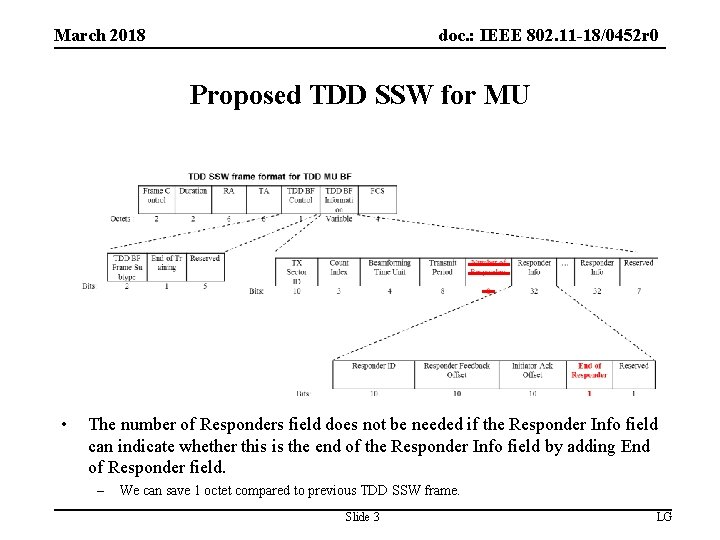doc. : IEEE 802. 11 -18/0452 r 0 March 2018 Proposed TDD SSW for MU • The number of Responders field does not be needed if the Responder Info field can indicate whether this is the end of the Responder Info field by adding End of Responder field. – We can save 1 octet compared to previous TDD SSW frame. Slide 3 LGdoc. : IEEE 802. 11 -18/0452 r 0 March 2018 Overhead analysis • Due to LDPC encoding process(block-wise encoding), even 1 octet can increase PPDU duration significantly. – Considering 32 spreading of control PHY and the number of training sectors, overhead becomes more severe. • There are factors which affect the overhead. – The number of responders – The number of training sectors • So, we investigate the overhead depending on the factors above. Slide 4 LGdoc. : IEEE 802. 11 -18/0452 r 0 March 2018 Overhead analysis (TDD slot duration) • When the number of responders is 11, 32, 53, 74, 95, 116, 137, 158, 179, 200, 221, and 242, proposed TDD SSW frame needs one less LDPC codeword for encoding compared to existing TDD SSW frame. • When the number of responders is 11. – TXTIME[TDD SSW for MU] of existing frame = 30. 56 us – TXTIME[TDD SSW for MU] of proposed frame = 27. 35 us Ø We can save about 3 us TDD slot duration per each TDD SSW frame. • When the TDD slot duration is longer than 113 us and less shorter than 125 us, – The maximum number of transmission of exiting TDD SSW frame is 3. – The maximum number of transmission of proposed TDD SSW frame is 4. Ø We can transmit one more TDD SSW frame. Slide 5 LGdoc. : IEEE 802. 11 -18/0452 r 0 March 2018 Overhead analysis (Number of responders) • The time cost of proposed frame is decreased when the number of responders is 11, 32, 53, 74, 95, 116, 137, 158, 179, 200, 221, and 242. Time Cost* (usec) Number of Responders Existing Proposed 10 19389. 71 19315. 00 74. 71 11 21772. 34 20128. 69 1643. 64 12 22586. 03 22511. 32 74. 71 31 42753. 03 42678. 31 74. 71 32 45135. 65 43492. 01 1643. 64 33 45949. 35 45874. 64 74. 71 *Assumption and configuration  : 16 TX Beams, and repeat TDD SSW frame 32 times for each TX Beam *Time Cost = {TXTIME[TDD SSW for MU]*32 + SBIFS*(32 -1) + TXTIME[TDD SSW Feedback]*N_Responders + TXTIME[TDD SSW ACK]*N_Responders + SBIFS*(N_Responders-1)} * 16 Slide 6 LG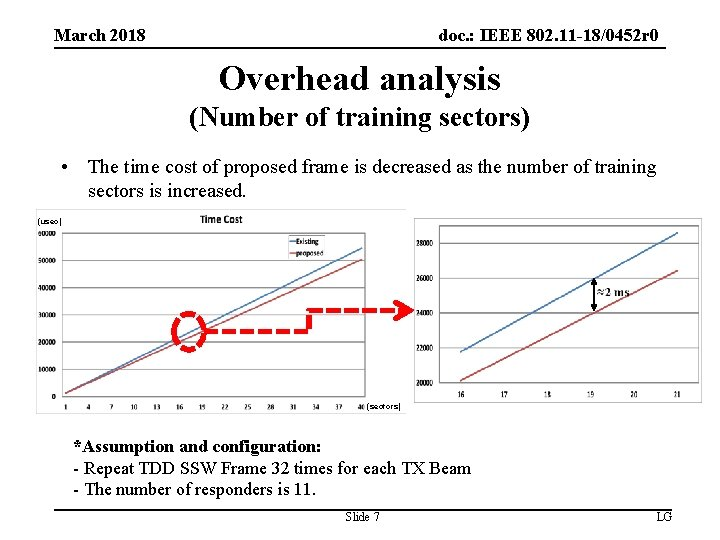doc. : IEEE 802. 11 -18/0452 r 0 March 2018 Overhead analysis (Number of training sectors) • The time cost of proposed frame is decreased as the number of training sectors is increased. (usec) (sectors) *Assumption and configuration: - Repeat TDD SSW Frame 32 times for each TX Beam - The number of responders is 11. Slide 7 LGdoc. : IEEE 802. 11 -18/0452 r 0 March 2018 Conclusion • A TDD SSW frame for MU improves time efficiency for a case in which the initiator perform BF training with multiple responders. • In terms of time efficiency, the existing TDD SSW frame for MU needs to be modified. • The proposed TDD SSW frame for MU can make the time cost reduced. Slide 8 LG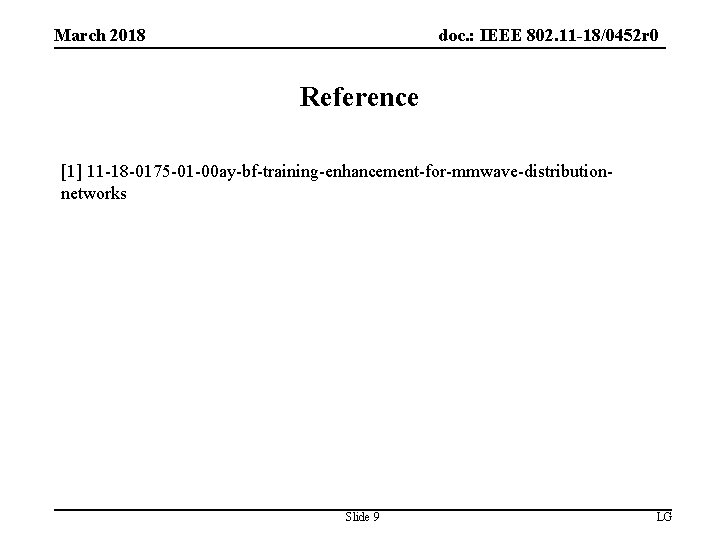doc. : IEEE 802. 11 -18/0452 r 0 March 2018 Reference  11 -18 -0175 -01 -00 ay-bf-training-enhancement-for-mmwave-distributionnetworks Slide 9 LG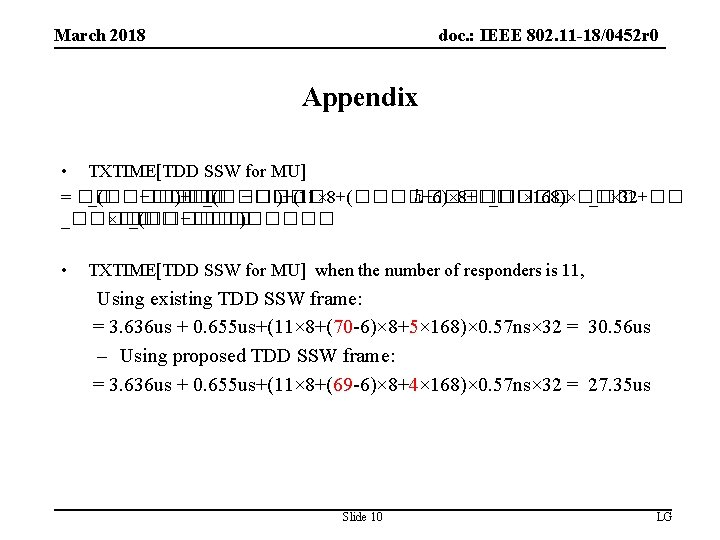doc. : IEEE 802. 11 -18/0452 r 0 March 2018 Appendix • TXTIME[TDD SSW for MU] = �� _(������ −���� )+�� _(���� −���� )+(11× 8+(����� ℎ− 6)× 8+�� _���� × 168)×�� _�� × 32+�� _������ ×�� _(������ −���� ) • TXTIME[TDD SSW for MU] when the number of responders is 11, Using existing TDD SSW frame: = 3. 636 us + 0. 655 us+(11× 8+(70 -6)× 8+5× 168)× 0. 57 ns× 32 = 30. 56 us – Using proposed TDD SSW frame: = 3. 636 us + 0. 655 us+(11× 8+(69 -6)× 8+4× 168)× 0. 57 ns× 32 = 27. 35 us Slide 10 LG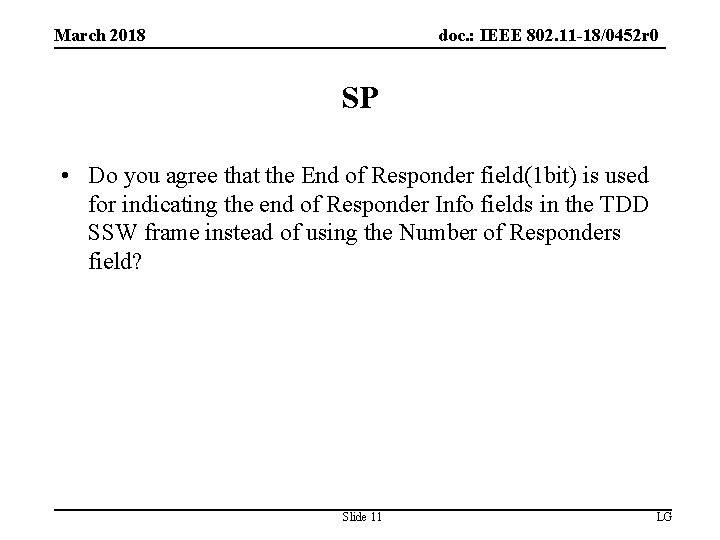doc. : IEEE 802. 11 -18/0452 r 0 March 2018 SP • Do you agree that the End of Responder field(1 bit) is used for indicating the end of Responder Info fields in the TDD SSW frame instead of using the Number of Responders field? Slide 11 LG